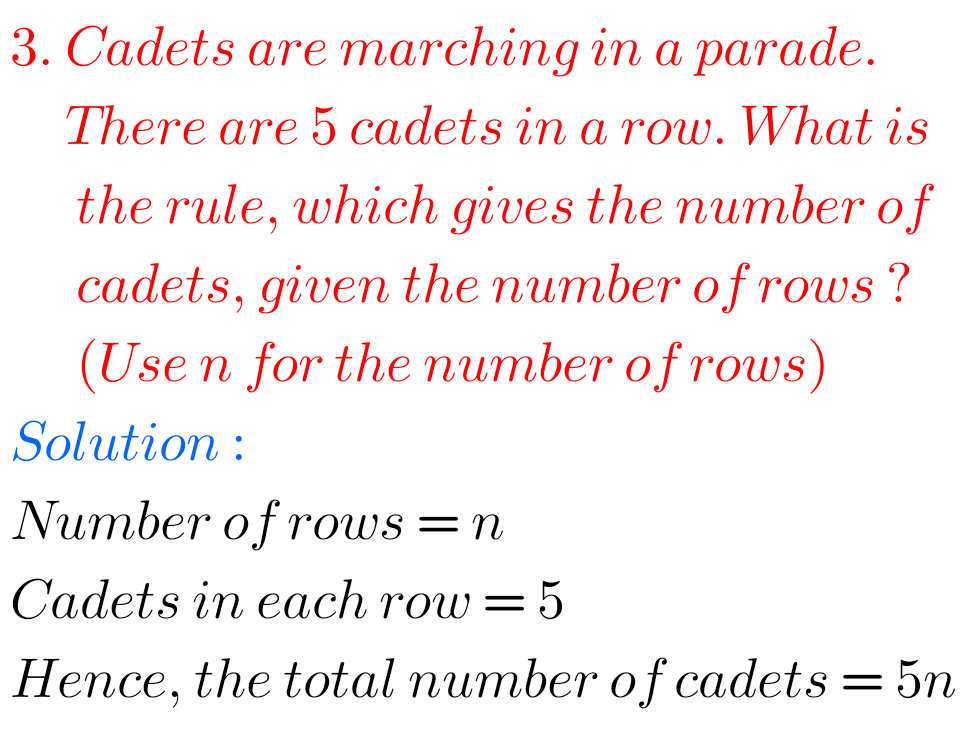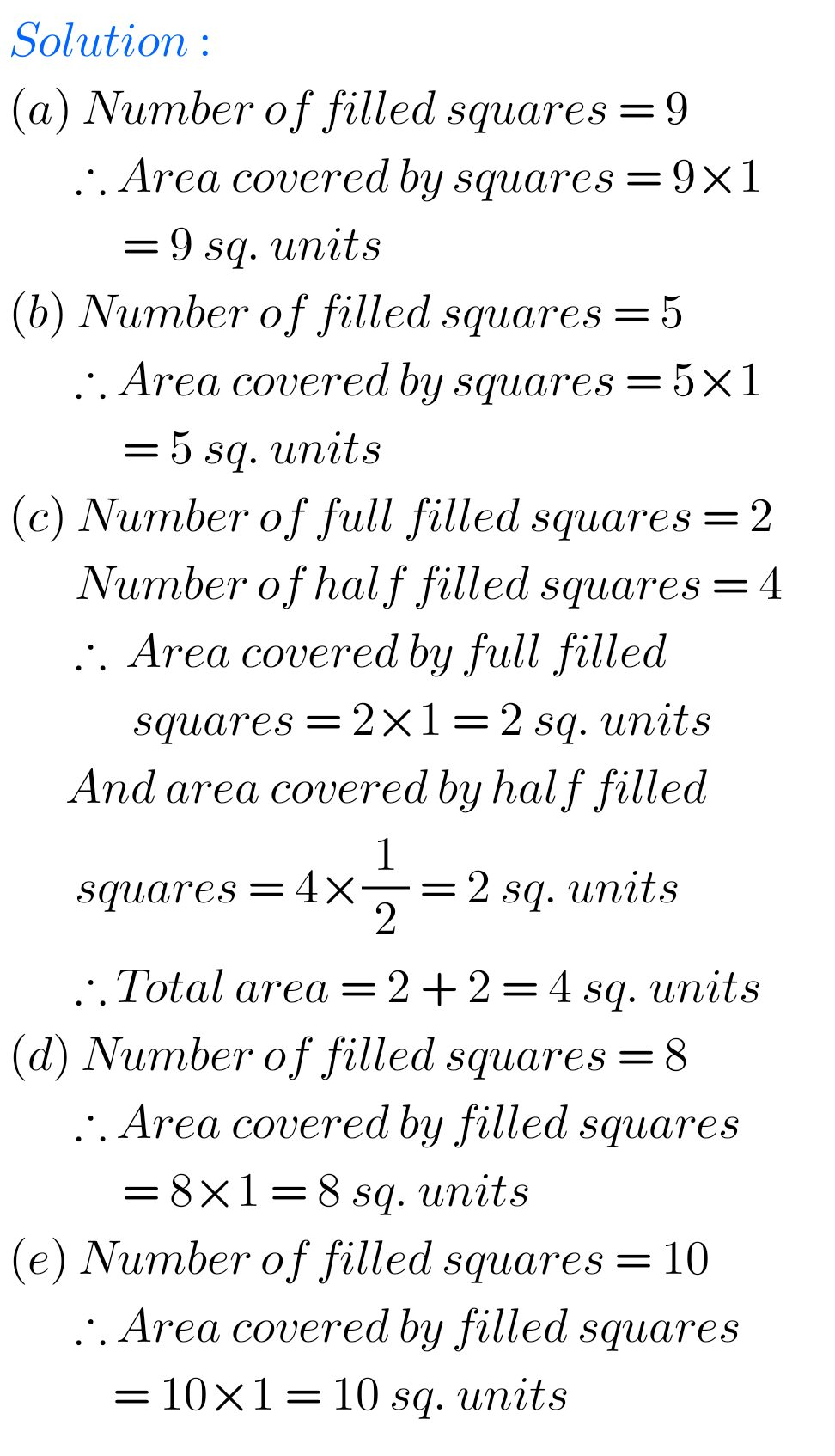# solutions for class 6 th mathematics## Ncert Maths Solutions for class 6

Maths solutions for class 6 NCERT NCERT Maths solutions for class 6 for some chapters are given. First you study the textbook lesson very well. You should also observe the example problems and solutions given in the textbook. Try them well. Observe the given below solutions and try them in your own method. Solutions for …## NCERT Maths solutions for Ratios and Proportions chapter 12 exercise 12.3 Class 6

Class 6 Chapter 12 exercise 12.3 solutions NCERT maths Ratios and Proportions NCERT maths class 6 chapter 12 Ratios and Proportions exercise 12.3 solutions are given. You should study the textbook lesson Ratios and Proportions very well. You should also observe, and practice example problems and solutions given in the textbook. Observe the given below …## NCERT solutions for maths class 6 chapter 12 exercise 12.2 Ratios and Proportions

Chapter 12 exercise 12.2 solutions NCERT maths class 6 Ratios and Proportions NCERT maths class 6 chapter 12 Ratios and Proportions exercise 12.2 solutions are given. You should study the textbook lesson Ratios and Proportions very well. You should also observe, and practice example problems and solutions given in the textbook. Observe the given below …

## NCERT solutions for maths class 6 exercise 12.1 Ratios and Proportions chapter 12

Ratio and Proportion Exercise 12.1 solutions NCERT Maths Class 6 NCERT class 6 Mathematics chapter 12 Exercise 12.1 Ratio and Proportion solutions are given. You should observe and practice all example problems and solutions given in the textbook. Observe the solutions and try them in your own method. NCERT solutions for maths Class 6 Ratios …

## Chapter 11 Algebra exercise 11.2 solutions Class 6  Ncert Maths

Algebra chapter 11 exercise 11.2 solutions NCERT Maths Class 6-chapter 11 Algebra exercise 11.2 solutions Maths NCERT textbook are given. First you should study the textbook lesson Algebra very well. You should also observe the example problems and solutions given in the solutions. Observe the solutions and try them in your own method. You can …## Ncert solutions for maths class 6 Algebra exercise 11.1 chapter 11

Maths class 6 chapter 11 exercise 11.1 NCERT solutions Class 6-chapter 11 Algebra exercise 11.1 solutions Maths NCERT textbook are given. First you should study the textbook lesson Algebra very well. You should also observe the example problems and solutions given in the solutions. Observe the solutions and try them in your own method. You …## Ncert solutions for Mensuration chapter 10 exercise 10.3 class 6

Class 6 chapter 10 Mensuration exercise 10.3 solutions Class 6 Maths NCERT Chapter 10 Mensuration Exercise 10.3 solutions are given. First you should study the textbook lesson Mensuration very well. You should also observe the example problems and solutions given in the textbook. Observe the given solutions and try them in your own method. You …## Mensuration Exercise 10.2 solutions chapter 10 class 6 ncert maths

NCERT solutions class 6 maths exercise 10.2 Mensuration Class 6 Maths NCERT Chapter 10 Mensuration Exercise 10.2 solutions are given. First you should study the textbook lesson Mensuration very well. You should also observe the example problems and solutions given in the textbook. Observe the given solutions and try them in your own method. You …## Ncert Maths solutions for Mensuration chapter 10 exercise 10.1 class 6

Mensuration solutions class 6 maths exercise 10.1 Class 6 Maths NCERT Chapter 10 Mensuration Exercise 10.1 solutions are given. First you should study the textbook lesson Mensuration very well. You should also observe the example problems and solutions given in the textbook. Observe the given solutions and try them in your own method. You can see. …

## Ncert maths solutions for class 6 chapter 9 exercise 9.1 Data Handling

Data Handling Solutions Class 6 Exercise 9.1 NCERT Maths Class 6 Maths NCERT Chapter 9 Data Handling Exercise 9.1 solutions are given. Study the textbook lesson Data Handling very well. Practice the example problems and solutions given in the textbook. Observe the given solutions and try them in your own method. NCERT class 6 maths …